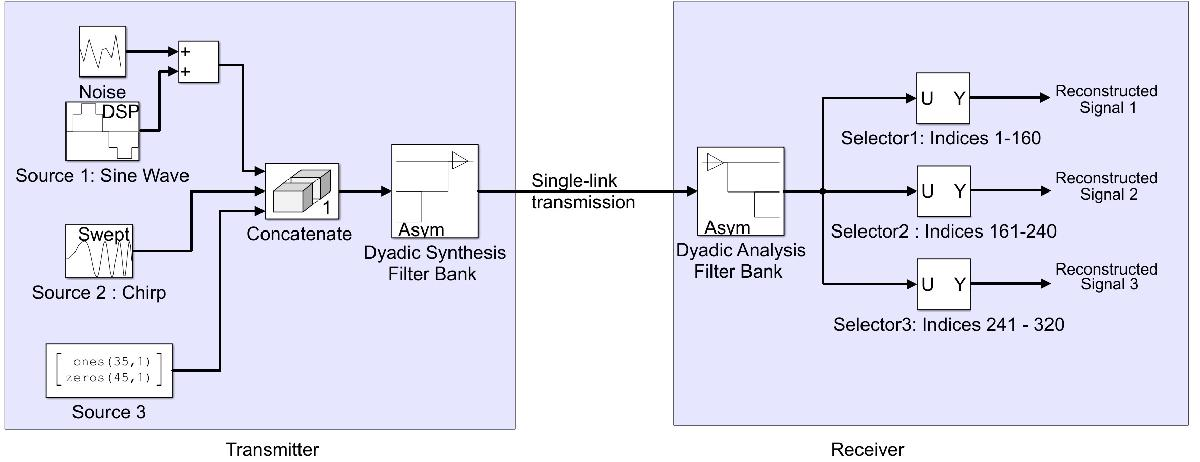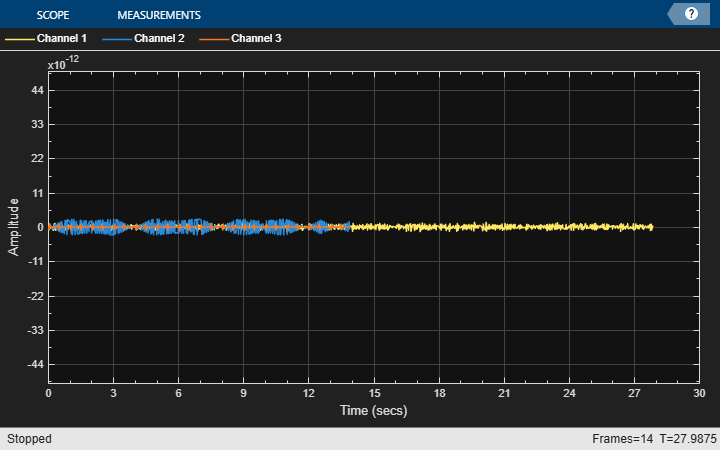# Three-Channel Wavelet Transmultiplexer

This example shows how to reconstruct three independent combined signals transmitted over a single communications link using a Wavelet Transmultiplexer (WTM). The example illustrates the perfect reconstruction property of the discrete wavelet transform (DWT).

### Introduction

This WTM combines three source signals for transmission over a single link, then separates the three signals at the receiving end of the channel. The example demonstrates a three-channel transmultiplexer, but the method can be extended to an arbitrary number of channels.

The operation of a WTM is analogous to a frequency-domain multiplexer (FDM) in several respects. In an FDM, baseband input signals are filtered and modulated into adjacent frequency bands, summed together, then transmitted over a single link. On the receiving end, the transmitted signal is filtered to separate adjacent frequency channels, and the signals are demodulated back to baseband. The filters also must strongly attenuate the adjacent signal to provide a sharp transition from the filter passband to its stopband. This step limits the amount of crosstalk, or signal leakage, from one frequency band to the next. In addition, FDM often employs an unused frequency band between the three modulated frequency bands, known as a guard band, to relax the requirements on the FDM filters.

In a WTM, the filtering performed by the synthesis and analysis wavelet filters is analogous to the filtering steps in the FDM, and the interpolation in the synthesis stage is equivalent to frequency modulation. From a frequency domain perspective, the wavelet filters are fairly poor spectral filters as compared to the filters required by a FDM implementation, exhibiting slow transitions from passband to stopband, and providing significant distortion in their response. What makes the WTM special is that the analysis and synthesis filters together completely cancel the filter distortions and signal aliasing, producing perfect reconstruction of the input signals and thus perfect extraction of the multiplexed inputs. Ideal spectral efficiency can be achieved, since no guard band is required. Practical limitations in the implementation of channel filters create out-of-band leakage and distortion. In the conventional FDM approach, each channel within the same communications system requires its own filter and is susceptible to crosstalk from neighboring channels. With the WTM method, only a single bandpass filter is required for the entire communications channel, while channel-to-channel interference is eliminated.

Note that a noisy link can cause imperfect reconstruction of the input signals, and the effects of channel noise and other impairments in the recovered signals can differ in FDM and WTM. This can be modeled, for example, by adding a noise source to the data link.

The following block diagram illustrates the reconstruction of three independent signals transmitted over a single communications link using a WTM.### Initialization

Creating and initializing your System objects before they are used in a processing loop is critical to get optimal performance. Initialize variables used in the example such as the standard deviation of the channel noise.

```load dspwlets; % load filter coefficients and input signal NumTimes = 14; % for-loop iterations stdnoise = .2^.5; % standard deviation of channel noise```

Create a sine wave System object™ to generate the Channel 1 signal.

```sine = dsp.SineWave(Frequency = fs/68, ... SampleRate = fs, ... SamplesPerFrame = fs*2);```

Create a random number generator stream for the channel noise.

`strN = RandStream.create('mt19937ar','seed',1);`

Create a chirp System object to generate the Channel 2 signal.

```chirpSignal = dsp.Chirp( ... Type = 'Swept cosine', ... SweepDirection = 'Bidirectional', ... InitialFrequency = fs/5000, ... TargetFrequency = fs/50, ... TargetTime = 1000, ... SweepTime = 1000, ... SampleRate = 1/ts, ... SamplesPerFrame = fs);```

Create and configure a dyadic synthesis filter bank System object for reconstructing the signal from different subbands of the signal.

```dyadicSynthesis = dsp.DyadicSynthesisFilterBank( ... CustomLowpassFilter = [0 lor], ... CustomHighpassFilter = [0 hir], ... NumLevels = 2 ); ```

Create and configure a dyadic analysis filter bank System object for subband decomposition of the signal.

```dyadicAnalysis = dsp.DyadicAnalysisFilterBank( ... CustomLowpassFilter = lod, ... CustomHighpassFilter = hid, ... NumLevels = 2 );```

Create three System objects for inserting delays in each channel to compensate for the system delay introduced by the wavelet components.

```delay1 = dsp.Delay(4); delay2 = dsp.Delay(6); delay3 = dsp.Delay(6);```

Create time scope System objects to plot the original, reconstructed and error signals.

```scope1 = timescope(Name = 'Three Channel WTM: Original (delayed)', ... SampleRate = fs, ... TimeSpan = 30, ... YLimits = [-2 2], ... ShowLegend = true, ... TimeSpanOverrunAction = 'Scroll'); pos = scope1.Position; pos(3:4) = 0.9*pos(3:4); scope1.Position = [pos(1)-1.1*pos(3) pos(2:4)]; scope2 = timescope(Name ='Three Channel WTM: Reconstructed', ... Position = pos, ... SampleRate = fs, ... TimeSpan = 30, ... YLimits = [-2 2], ... ShowLegend = true, ... TimeSpanOverrunAction = 'Scroll'); scope3 = timescope(Name = 'Three Channel WTM: Error', ... Position = [pos(1)+1.1*pos(3) pos(2:4)], ... SampleRate = fs, ... TimeSpan = 30, ... YLimits = [-5e-11 5e-11], ... ShowLegend = true, ... TimeSpanOverrunAction = 'Scroll'); Tx_Ch3 = [ones(35,1);zeros(45,1)]; % Generate the Channel 3 signal```

### Stream Processing Loop

Create a processing loop to simulate the three channel transmultiplexer. This loop uses the System objects you instantiated above.

```for ii = 1:NumTimes Tx_Ch1 = sine() + ... stdnoise*randn(strN,fs*2,1); % Generate Channel 1 signal Tx_Ch1_delay = delay1(Tx_Ch1); Tx_Ch2 = chirpSignal(); % Generate Channel 2 signal Tx_Ch2_delay = delay2(Tx_Ch2); Tx_Ch3_delay = delay3(Tx_Ch3); % Delayed Channel 3 signal % Concatenate the three channel signals Tx = [Tx_Ch1; Tx_Ch2; Tx_Ch3]; % Synthesis stage equivalent to frequency modulation. y = dyadicSynthesis(Tx); % Analysis stage Rx = dyadicAnalysis(y); % Separate out the three channels Rx_Ch1 = Rx(1:160); Rx_Ch2 = Rx(161:240); Rx_Ch3 = Rx(241:320); % Calculate the error between TX and RX signals err_Ch1 = Tx_Ch1_delay - Rx_Ch1; err_Ch2 = Tx_Ch2_delay - Rx_Ch2; err_Ch3 = Tx_Ch3_delay - Rx_Ch3; % Plot the results. scope1(Tx_Ch1_delay, Tx_Ch2_delay, Tx_Ch3_delay); scope2(Rx_Ch1, Rx_Ch2, Rx_Ch3); scope3(err_Ch1, err_Ch2, err_Ch3); end release(scope1);````release(scope2);``release(scope3);`### Summary

In this example, you used the `DyadicAnalysisFilterBank` and `DyadicSynthesisFilterBank` System objects to implement a Wavelet Transmultiplexer. The perfect reconstruction property of the analysis and synthesis wavelet filters enables perfect extraction of multiplexed inputs.